• Supervised learning : no matter what algorithm, more importance is get lot of data, and choosing wisely which features to be incoporated, regularization etc.
• One of most powerful SL; SVM: compared to logistic, neural networks, more powerful  non-linear function.
• Use in many industries. Last supervised learning algorithms that spent lot amount of time by Andrew Ng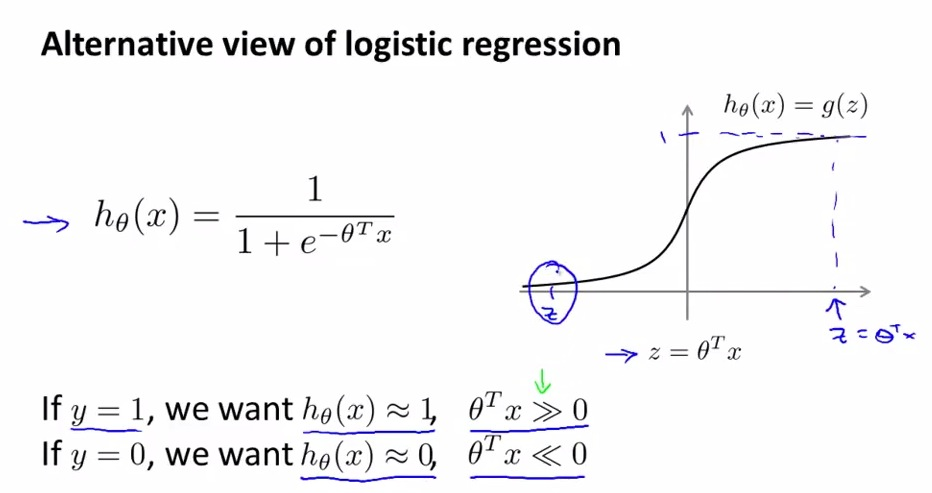• SVM : modified logistic regression
• The hypothesis hopes to approach the actual output; the value of z must be much larger than zero. By the value much much larger than zero, then the hypothesis will be approaching 1. And works the other way around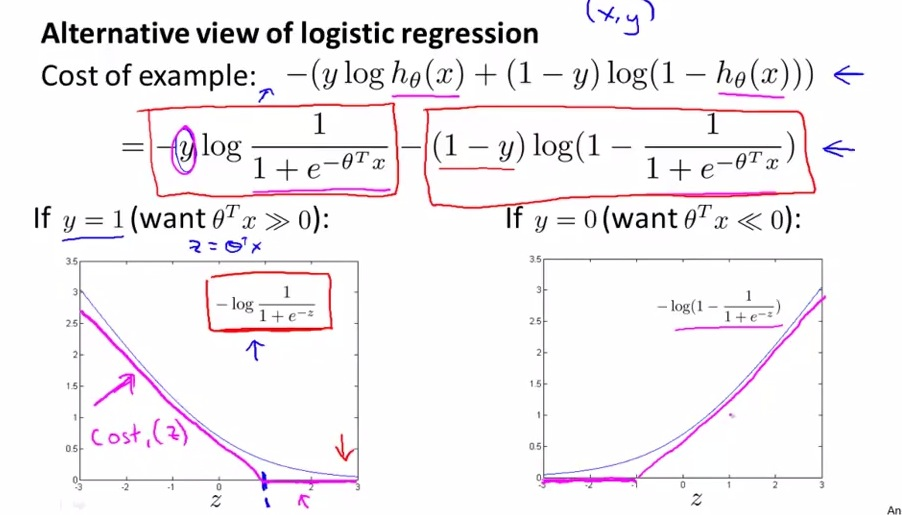• Remember that these alternative view is trying to nullify each other depends on whether y  == 1 or y == 0
• The formula above, is when we are using 1 training example. Notice the z value of 1, that is a joint point between slanted line and flat line.
• In logistic regression, with y =1, as the cost function approach zero(approach actual output), the z becomes much larger than zero.
• And on the bottom right, with y = 0 as the cost function approach zero(approach actual output), the z becomes much smaller than zero.
• In using SVM, we modified the logistric regression to be linearity. Using two line, slate going down to right, and flat right. By doing these, we are giving SVM a big advantage and much easier to implement.
• With gaining 2 linear formula denotes by cost1(z) and cost0(z), we are preparing to implement SVM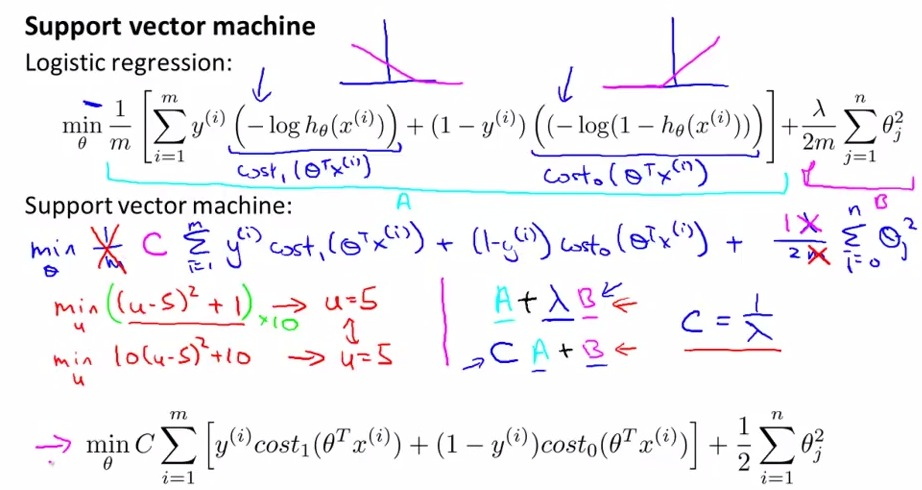• For SVM, get rid of 1/m, this is the other convention made for SVM. that is if we get rid m, that the features won't likely to change.See the examples in red for more intuition
• For the other convention, we simplify the equation with denotes the cost function with A, and regularization term with B.
• Now we''re modifying, the other convention to be just like the simple term on the right.
• lambda nullified, instead we are using c term.
• Is not that c = 1/lambda. But it posses equal result to lambda.
• The c value is really small so that B can have higher weight than cA. We don't weight B anymore, but instead makes A much more lighter. This way, we still trying to minimize the cost function.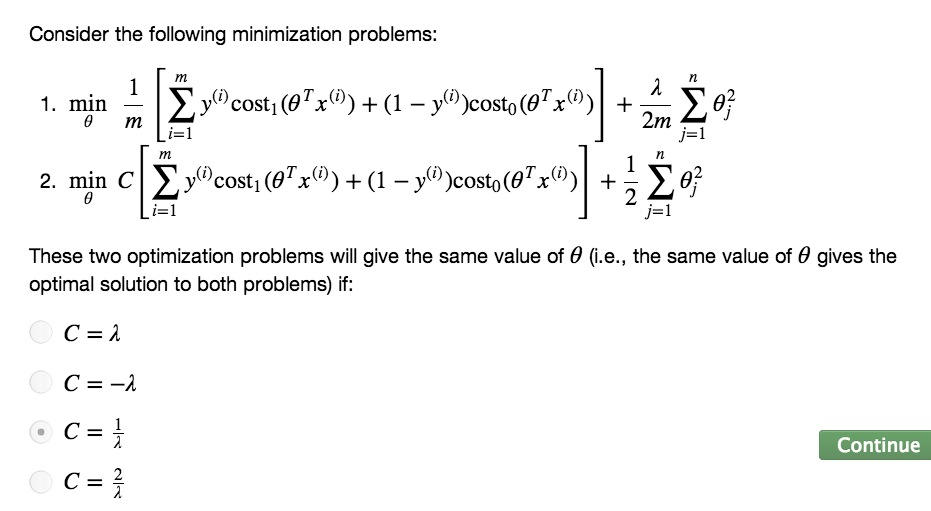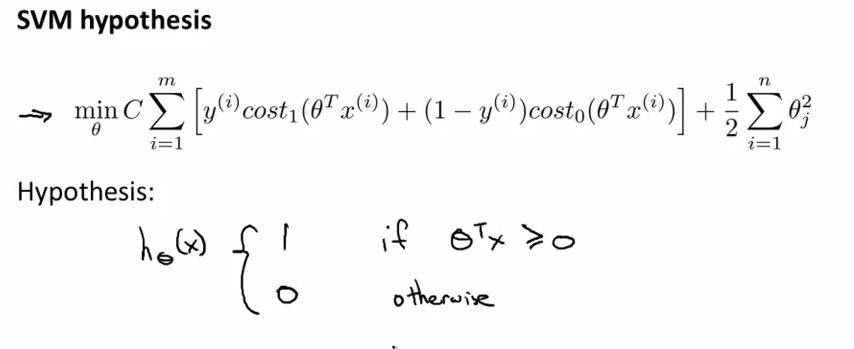• These are mathematical definition in SVM
• Differ from logistic regression, SVM doesn't count probability anymore. Instead we are using either 1 or 0 (not range from 0 to 1 like in logistic) for condition stated above
• Next, what hypothesis SVM result, dig more about Optimization Objective, and add a little bit to manage more complex non-linear function.